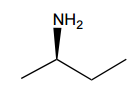Start typing, then use the up and down arrows to select an option from the list.# Organic Chemistry

Learn the toughest concepts covered in Organic Chemistry with step-by-step video tutorials and practice problems by world-class tutors.

15. Analytical Techniques:IR, NMR, Mass Spect

# 1H NMR:Number of Signals

1

#### concept

General Assumption for 1H NMR Signals4m
Play a video:
2

#### example

Identifying Proton Signals1m
Play a video:
Alright, guys. So what was the answer for question? Be three. Let's go ahead and check it out. First of all, did you guys find any symmetry in this molecule? Actually, yes. This is a molecule with a plane of symmetry down the middle. That means that whatever conclusion I make, let's say about this carbon over here also applies to the one across from it meaning that if you are able to identify the amount of unique hydrogen on one side that dotted line, the same exact thing applies to the other side. So you don't even count the ones on the other side. Okay, So for example, I noticed that this one is on a double bond. So I'm gonna make this as hydrogen type a. Then I notice that these hydrogen are on an al cane. A regular SP three hybrids. Carbon. That's gonna be another type of hydrogen. Now, I also noticed that there's this carbon here. I'm wondering, Did you guys give that a signal or not? Actually, this carbon doesn't even count because that carbon doesn't have any hydrogen. Remember, this is called proton NMR because it on Lee responds to proton. So even though that is a unique position on the molecule. It doesn't have hydrogen, so we don't count it. Then finally we have Over here, we have the hydrogen that are on that one. So that's its own unique place. Okay, so we've got those three different signals now. Would we have to also draw the signals on the other side? No. Because this one is also A This one is also be, And this one is also see. Okay, so that's what you do with the plane of symmetry. It means that any conclusions you have about one side are going to be the same exact ones on the on the next side. Okay, awesome. So three different ones. Let's go ahead and move on to the next question.
3

#### example

Identifying Proton Signals1m
Play a video:
and the answer for problems. See Iwas. Just one. Okay, so I know some of you guys got that because it turns out, first of all, is there any symmetry? Actually, yes. There's actually two planes of symmetry. There's a plane of symmetry here. I'll make that the red plane. And there's also plane of symmetry here. I'll make that the blue plane. Okay. Now, what does it mean to have a plane of symmetry again? It means that basically whatever you figure out for one side of the mirror applies to the other. Okay, in this sides, in this case, since I have two overlapping planes of symmetry, that means all I need to do is figure out the number of unique positions in one quadrant and that will apply to all the other three. Okay, So all I need to figure out is how many different positions do I have in one quadrant? Okay. And what we notices in that one quadrant, we have only two different positions. We have the red position and we have the blue position. Okay, so my question is, which of these get signals. Okay, well, red notice. Red actually already has four bonds so red isn't gonna count. Red isn't going to get a signal. Now, Blue is going to get a signal. But notice that this blue is the same as this one. It's the same groups trying to use blue here. It's the same as this one. This one, This one It's also the same. Is these okay? Those are all the same. So the answer is that you're just going to get one. So I'm just gonna put the letter A Because there's nothing else. Okay, awesome. Next question.
4

#### example

Identifying Proton Signals2m
Play a video:
So what was the answer that you got for D? Well, this one's actually kind of a high number. I want to start off by just talking about symmetry. Were you able to find any symmetry on this molecule if you did, you were kind of like deluding yourself because there actually isn't. This is an asymmetrical molecule. Now it would actually be easy to turn it into a symmetrical molecule if you didn't have this part. Let's say this Ethel Group didn't exist. That would be symmetrical, because now we would have a dotted line down the middle. And now anything that I determined for one half applies to the other. But that's not the molecule I gave you. I gave you a molecule that the two alcanzar symmetrical. But then one side has an ethyl on the other side. Doesn't that means there's no symmetry that literally means that every single atom needs its own signal. So I'm going to get Adam a signal. Be signals. See signal D signal E signal F signal G on signal age. Okay, so all of those were going to get their own unique signal. It's eight signals now. You might be wondering, guys, I know a few of you guys were wondering Johnny, Why did you give a and F different signals? They looked just the same to me. Okay. Why would you give them different signals? Well, notice F is closer to the Ethel Group, and A is further from the Ethel group. So that means there isn't perfect symmetry here, Right? If the Ethel group didn't exist, then A and F would have been the same thing. But since that Ethel Group is there, there's no symmetry. That means that F and A are their own unique peaks. Okay, so hopefully that makes sense. So far, let's move on to the next part.
5
Problem

How many types of electrically unique protons (peaks) are there in the following molecule?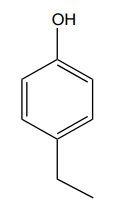6
Problem

How many types of electrically unique protons (peaks) are there in the following molecule?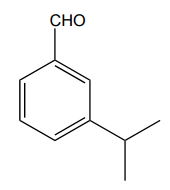7
Problem

How many types of electrically unique protons (peaks) are there in the following molecule?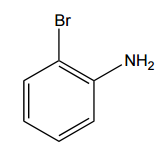8
Problem

How many types of electrically unique protons (peaks) are there in the following molecule?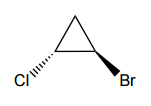9
Problem

How many types of electrically unique protons (peaks) are there in the following molecule?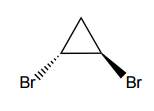10
Problem

How many types of electrically unique protons (peaks) are there in the following molecule?